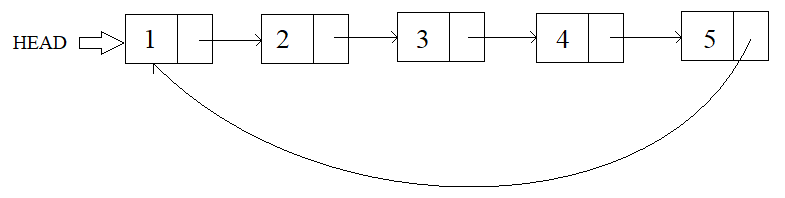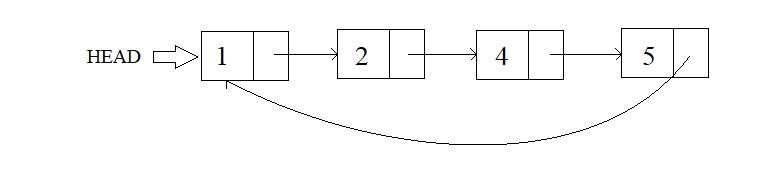3

# Deletion In Circular Linked List

Difficulty: EASYContributed By
Prateek Kalyani
Avg. time to solve
30 min

Problem Statement
Suggest Edit

#### You have to write a function that finds the given key in the list and deletes it. If no such key is present, then the list remains unchanged.

##### For Example :
``````This is a visualization of the Circular Linked List, represented by:
1 2 3 4 5 -1
``````##### Note :
``````The Circular Linked List before/after deletion may happen to be empty. In that case, only print -1.

All integers in the list are unique.
``````

##### Input format :
``````The first input line contains the integers present in the circular linked list in order.

The second input line contains a single integer 'key', the key to be deleted.
``````
##### Remember/Consider :
``````-1 marks the end of the linked list however, the tail of the linked list would be pointing to the head making it circular in nature.
``````
##### Output format :
``````The only output line contains the updated circular linked list post deletion.
``````
##### Constraints :
``````0 <= N <= 10 ^ 5
1 <= key <= 10 ^ 5

Where 'N' is the length of the Circular Linked List.

Time limit: 1 sec
``````
##### Sample Input 1 :
``````1 2 3 4 5 -1
3
``````
##### Sample Output 1 :
``````1 2 4 5 -1
``````
##### Explanation for Sample 1 :
``````The given linked list, before deletion:
````````````After deletion :
``````##### Sample Input 2 :
``````1 2 3 4 5 -1
1
``````
##### Sample Output 2 :
``````2 3 4 5 -1
``````Console